# Geometría proyectiva: Overlapping series of second order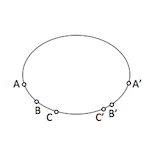When the base of a series is a conic the series are of second order.

As in the case of series of first order when we defined the overlapping series, we can establish proyectividades between two sets of second order with the same base (in this case a conical).

The process of working with this series is similar to that we saw obtain homologous elements in the two series proyectividades between first order, in which we determined intermediate outlook forms (straight beams) determining its perspective axis denominábamos “projective axis series“.

The projectivity between two overlapping series second order will be determined when we know three pairs of homologous points located on the same conical. (A-A’, B-B’, C-C’)

Recall that a cone will be determined by five conditions (the tangent points straight). As additional comments, Remember that a straight line is determined by two of your points, But if we want to define an is between overlapping series we need to relate three pairs of points that belong to the straight.

In Figure, projectivity is defined by the pairs of homologous points A-A ', B-B’ y C-C’.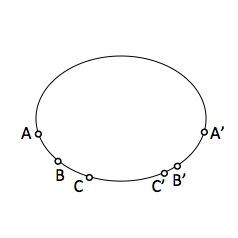If we project from two homologous points (for example the A and A ') the elements of each series are obtained perspectival do as they have a double beam (a-a’). These beams will be cut on your perspective axis will be the “projective axis of the series of second order”. This straight line is known by the nickname of “Straight from Pascal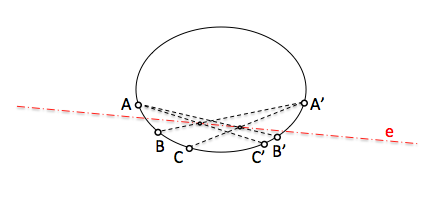To determine the homologue element of a point X either operate as with first-order series. X will project the point from an item (el A’) for you to obtain the associated ray beams above perspectival. The ray beam is cut counterpart in the perspective axis beams (projective axis series) and contain the point X’ X homolog,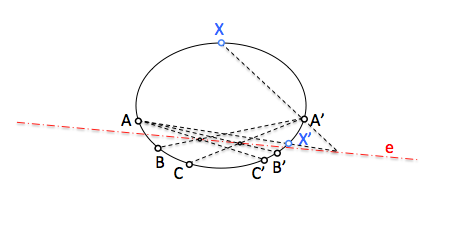The cutoff points of the projective axis determine the double elements of the overlapping series of second order. To check this, obtain the counterpart considering these points belong to any series, as we have done with the above point X transformed. The reader is left checking.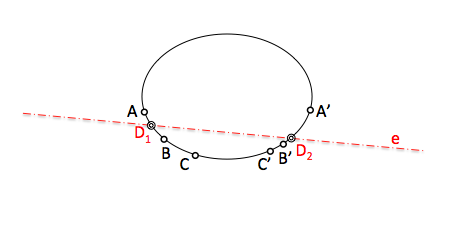Note that this analysis is shown tapered to improve understanding of the concepts. As the conical not we generally, obtaining X element’ homolog of X must be determined by the intersection of two lines by repeating the procedure of a new vertex projection.

However see that they are especially useful when the overlapping series is a circumference tapered, and having the same treatment but if the curve will be present in our paths and may be used.

Projective Geometry